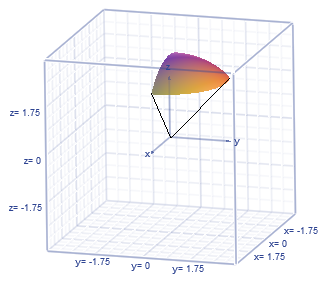# Sketch the solid whose volume is given by the integral below and evaluate the integral....

## Question:

Sketch the solid whose volume is given by the integral below and evaluate the integral.

{eq}\displaystyle \int^{\frac{\pi}{4}}_0 \int^\pi_0 \int^3_0 \rho^2 \sin (\phi)\ d \rho\ d \theta\ d \phi {/eq}.

## Spherical Coordinates:

Note that the integral above describes the volume of a spherical solid. We know this because the integrand is simply the differential volume element for spherical coordinates. We will plot the region then evaluate the integral to find the volume. Recall

{eq}x=\rho \cos \theta \sin \phi {/eq}

{eq}y = \rho \sin \theta \sin \phi {/eq}

{eq}z = \rho \cos \phi {/eq}

{eq}\rho^2 = x^2+y^2+z^2 {/eq}

{eq}\sqrt{x^2+y^2} = \rho \sin \phi {/eq}

{eq}dV = \rho^2 \sin \phi \ d\rho \ d\phi \ d\theta {/eq}

Let's take a moment to think about what this integral actually is. First, we recognize the integrand as the differential volume element for spherical coordinates, so we know the integral describes the volume of a spherical region. Note that the bounds on {eq}\phi {/eq} take us from the positive {eq}z {/eq}-axis all the way around to the negative {eq}z {/eq}-axis. Then {eq}\theta {/eq} takes us from the positive {eq}x {/eq}-axis to the line {eq}x=y {/eq} (i.e. the middle of the first quadrant). Lastly, the bounds on {eq}\rho {/eq} tell us that the sphere has radius 3. So we are dealing with a slice/wedge in the first octant. A sketch is below.The volume of the wedge is

{eq}\begin{align*} \int^{\pi/4}_0 \int^\pi_0 \int^3_0 \rho^2 \sin \phi\ d \rho\ d \theta\ d \phi &= \int_0^{\pi} d \theta \int_0^{\pi/4} \sin \phi\ d\phi \int_0^3 \rho^2\ d\rho \\ &= \pi \left [ -\cos \phi \right ]_0^{\pi/4} \left [ \frac13\rho^3 \right ]_0^3 \\ &= \frac\pi3 \left( - \frac{\sqrt2}{2} + 1 \right)(3^3) \\ &= \frac{9\pi}{2} \left( 2-\sqrt2 \right) \\ &\approx 8.2814 \end{align*} {/eq}